# Moment of inertia, definition, formula, moment of inertia for point mass, continuous mass system, practice problems, FAQs

We all know that the effort required to rotate a wheel with the axis in the middle is very less as compared to flipping the wheel against its axis. One might wonder what made the flipping difficult for the same wheel that can easily rotate. The reason behind this can be discussed only when one has knowledge about the moment of inertia (resistance to change).

• Moment of inertia
• Moment of inertia of point mass system
• Moment of inertia for a continuous body
• Moment of inertia of a uniform rod
• Moment of inertia of a uniform ring
• Practice problems
• FAQs

Moment of inertia

The analogue of mass in rotational motion is known as the moment of inertia. This is a quantity that offers the resistance to change its state of rest or uniform rotation of a body.• A moment of inertia is always positive like mass.
• It is a scalar quantity, the magnitude of which is directly proportional to the square of the distance between the mass and the axis of rotation.
• We cannot define a moment of inertia without an axis.
• Moment of inertia is also known as the second moment of mass.

The moment of inertia of a body depends on the following factors:

• Axis of rotation
• Shape and size of the body
• Density of the material of the body
• Distribution of mass relative to the axis of rotation

Amongst the given factors, the most important is the distribution of mass relative to the axis of rotation.

Moment of inertia of point mass system

Moment of inertia () of a particle of mass m located at distance r from an axis is given by the following: = mr2For multiple point mass system

We can add moments of inertia of multiple masses if they are defined with respect to the same axis of rotation. Moment of inertia of n particles of masses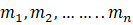at a distance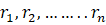, respectively, from an axis OO’ is given by the following: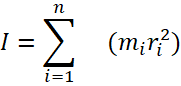Moment of inertia for a continuous body

In general, for a uniform body having a mass element dm having the moment of inertia dI,We can write the moment of inertia of the element as,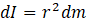By integrating the given formula, we get the moment of inertia of the entire body as,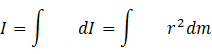To find the moment of inertia of the continuous bodies, its shape and size should be defined.

Let us now discuss the moment of inertia of different shapes of solids about the given axis.

Moment of inertia of a uniform rod

The moment of inertia of a uniform rod of mass M and length L is about an axis passing through its centre and perpendicular to the length.

Consider the linear mass density (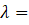mass per unit length) for a differential mass element dm as shown in the following figure:Differential mass element dm is at distance x from the axis of rotation OY.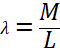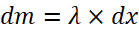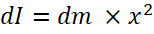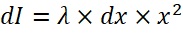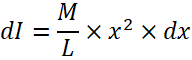Now, integrating within the limits -L/2 to L/2, we get,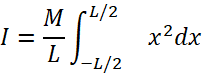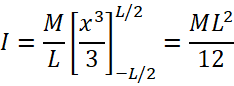Now, consider another case where the axis of rotation is at one of the ends of the rod.

Consider the linear mass density for a mass element dm as shown in the figure. The mass element dm is written in terms of linear mass density, and thickness dx is situated at a distance x from the axis of rotation OY.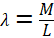So, by integrating dI between the limits 0 to L, we arrive at our final result for the moment of inertia of a rod having the axis of rotation at one of the ends.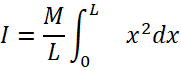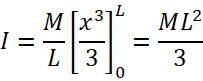Moment of Inertia of a Uniform Ring

The moment of inertia of a uniform ring of mass M and radius R about the centroidal axis

is perpendicular to the plane of the ring.Here, R represents the distance of the mass element dm from the axis of rotation.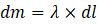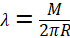and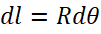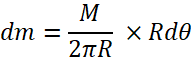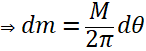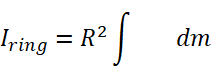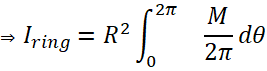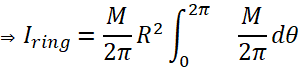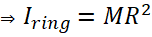Practice problems

Q. Five particles, each of mass 2 kg, are placed on a circular path as shown. Find the moment of inertia of the system about an axis passing through the centre of the circle and perpendicular to its plane.Solution: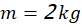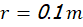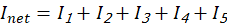As all the particles are of the same mass and at the same distance from the centre of rotation,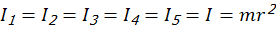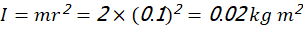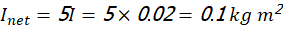Q. Three particles, each of mass m, are situated at the vertices of an equilateral triangle PQR of side a as shown in the figure. Find the moment of inertia of the system about the x-axis.Solution

We know that the moment of inertia of n particles of masses m1, m2,……..mnat a distance r1, r2……..rn, respectively, from an axis is given by the following:Here, three particles, each of mass m, are located as shown in the figure.Thus, the moment of inertia of the system aboutthe x-axis is the following:

Moment of inertia of system about x-axis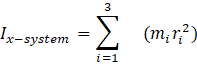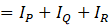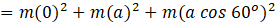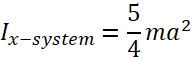Q. Two identical rods are joined to form an X. The smaller angle between the rods is θ. The moment of inertia of the system about an axis passing through the point of intersection of the rods and perpendicular to their plane is proportional to which of the following?

1. θ
2. sin2θ
3. cos2θ
4. Independent of θ\Solution

For an axis passing through the centre, the moment of inertia for both the rods is,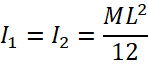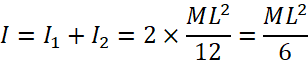This proves that the moment of inertia in this particular case is independent of θ.

Thus, option (D) is the correct answer.

Q. A half ring of mass 5 kg and radius 2 m has the moment of inertia around its natural axis as ____ .(A)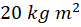(B)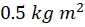(C)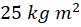(D)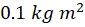Solution:

Consider this ring as a full ring folded to make a half ring.

The radius and the mass of this half ring will be the same as that of the full ring.

So, the moment of inertia will also be the same.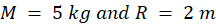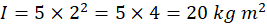Thus, option (A) is the correct answer.

FAQs

Q. A circular disc is to be made by using iron and aluminium so that it acquires the maximum

moment of inertia about its geometrical axis. It is possible with which of the following conditions?

1. The aluminium is in the interior and the iron is surrounding it.

(B) The iron is in the interior and the aluminium is surrounding it.

(C) The iron and aluminium layers are used in alternate order.

(D) The sheet of iron is used at the external surface and the sheet of aluminium is used as the internal layer.

Sol. If a body has more mass near the axis of rotation, then it will have a lower moment of inertia than the body having more mass distributed away from the axis of rotation.

In this case, to have a higher moment of inertia, it needs to have more mass distributed away from the axis of rotation that is passing through the centre.

The density of iron is greater than the density of aluminium. Therefore, iron rings need to be placed at the outer part of the disc.

Thus, option (A) is the correct answer.

Q. What is the dimensional formula for moment of inertia?

Sol.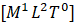Q. How is the moment of inertia of a body different from its mass?
Sol.
Mass of a body is a constant quantity, whereas the moment of inertia of a body depends upon its mass, size, shape, position and orientation, axis of rotation, distribution of mass.

Q. What is the physical significance of moment of inertia?
Sol.
Moment of inertia plays the same role as is played by mass in translatory motion. In translatory motion, mass is a measure of inertia, therefore, moment of inertia is a measure of rotational inertia in rotatory motion.

Talk to our expert
By submitting up, I agree to receive all the Whatsapp communication on my registered number and Aakash terms and conditions and privacy policy